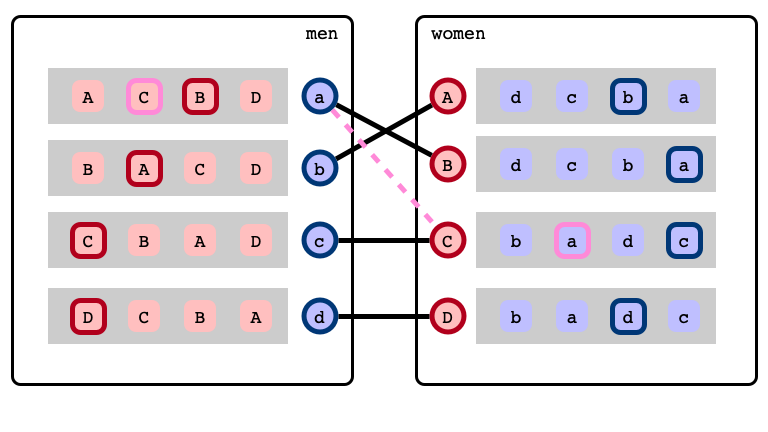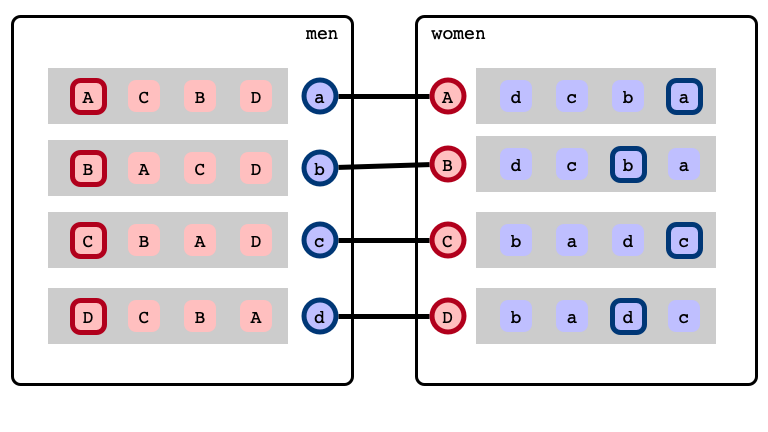# Stable Matchings with Restricted Preferences: Structure & Complexity

Christine Cheng (University of Wisconsin, Milwaukee)

Will Rosenbaum (Amherst College)

## Stable Marriage Problem## Stable Marriage Problem

A stable matching## Gale-Shapley Algorithm

Gale & Shapley 1962:

• Efficient algorithm that finds a stable matching
• stable matchings always exist!

## Intractible Problems

• How many stable matchings does an instance have?
• Can we find fair stable matchings?
• median
• balanced
• sex-equal

## Restricted Preferences

We consider restricted preference models:

1. $k$-bounded
2. $k$-attribute
3. $(k_1, k_2)$-list
4. $k$-range

2–4 introduced by Bhatnagar et al. (SODA 2008)

## Main Results

1. Characterize structure of stable matchings for preference models
2. $k$-bounded, $k$-attribute, $(k, \infty)$-list give generic structure
• intractible structural problems remain intractible
3. $k$-range instances have highly restricted structure
• fast algorithms for many problems